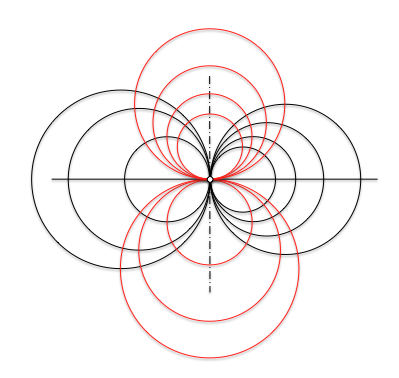Metric geometry : Make circles parabolic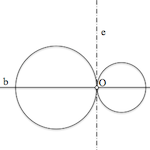When you define a beam circumferences infinity as a set simply fulfilling a restriction based on the power, sorted the beams depending on the relative position of its elements.

Los parabolic beams circumferences These families are among circumferences. We will see how to determine elements that belong.

Given the circles tangent to a point O, the radical axis “and” circumferences coincides with the common tangent to two circles. This line is perpendicular to the one containing the centers of the circumferences.

The endless circles tangent two circles tangent to each other at a point O, determine one parabolic beam circumferences. The punto O called beam center.

The radical axis of any two circles of this bundle is the line and.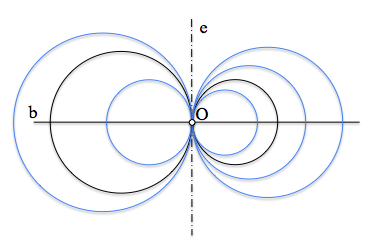All centers of the circumferences of the bundle are in a straight, b, denominada straight beam foundation.

Determine a circumference of the parabolic beam passing through a point P

From the endless circles of parabolic beam, only passes through a given point than the center O beam. Let's see how to determine the center of a circle of the beam passing through a point P any.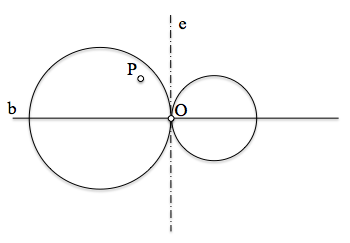The circumference sought O1 will be centered in the base line, b, and pass through the points P and O, so shall also the bisector of these points.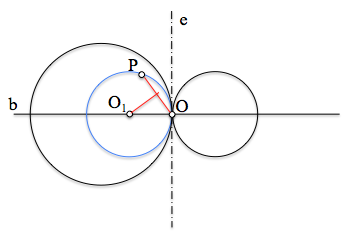Solution, its center, thus determined by the intersection of two loci, the base line and the bisector of the PO segment containing two crossing points.

Determine the parabolic beam circumferences are tangent to a given line

Tangency condition is determined by a straight t anyone who does not match with right or basis b or the radical axis and.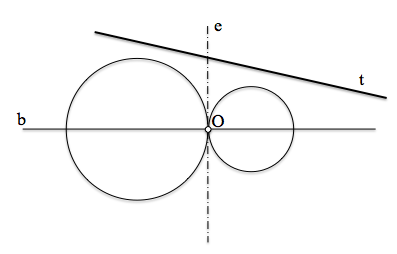To solve the problem look for a point Cr, radical shaft and, have equal power with respect to the beam circumferences, and belonging, and the embroidery, to the line t ya the latter is the center of the circumferences radical which are tangent. We see, that Cr is the radical center of the line t (infinite radius circumference) and parabolic beam circumferences.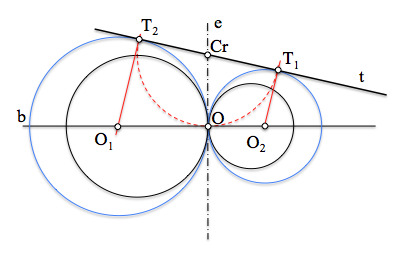As shown in Figure, power Cr on all beam circumferences finding can determine the distance (squared) center O beam. This distance is to be also the points of tangency of the solutions sought. We have two solutions because we can take this away Cr-O on both sides of Cr on the line t.

Determine the parabolic beam circumferences are tangent to a given circle

The generalization of the problem comes when the condition of tangency is on a circle t any.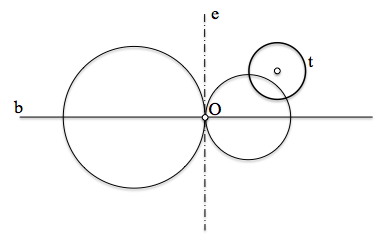In this case, again, determine a point Cr have equal power with respect to the circumference marking the tangency condition and any of the parabolic beam, so it must be in its radical axis.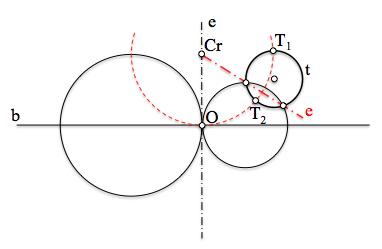The solutions pass through the points T1 and T2 located on tangents drawn from Cr, and which are at the root of the distance power that we have calculated as in the previous case.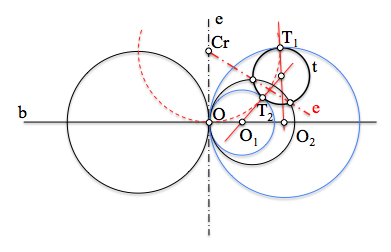The centers of the solutions found aligned with the center of the circle t and corresponding contact points.

Make conjugate

Last, we can see in the figure below the conjugate beam (orthogonal) of a parabolic beam, it can be deduced that is another parabolic base line the radical axis of the front.